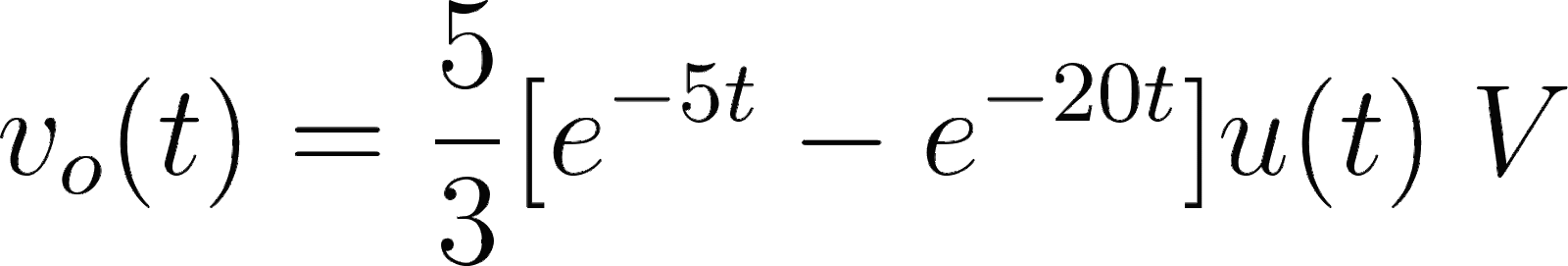Introduction To Electric Circuits - 9 Edition - Chapter 14.1 - Problem P14.10-7
Register Now

Join StudySoup

Get Full Access to Introduction To Electric Circuits - 9 Edition - Chapter 14.1 - Problem P14.10-79781118477502

# Solution: The input to a circuit is the voltage vs. The output is the voltage vo. The

Introduction to Electric Circuits | 9th Edition

Problem P14.10-7

The input to a circuit is the voltage vs. The output is the voltage vo. The step response of the circuit is vo t 5 3 e5t e20t u t V Determine the steady-state response of the circuit when the input is vs t 12 cos 30 t V

Accepted Solution
Step-by-Step Solution:

Step 1 of 4

Steady-state response of the circuit is,###### Chapter 14.1, Problem P14.10-7 is Solved

Step 2 of 4

Step 3 of 4

Unlock Textbook Solution